## Switching characteristic of Si MOSFET/GaN FET and diode

##### Introduction

The objective of this experiment is to study the switching characteristics of power MOSFETs and power diodes using a buck converter. The circuit will be operated in open loop conditions (no feedback). The main goal is to understand the switching behavior of these two power devices.

##### Theoretical background

The fundamental building block of all the converters studied in this lab is the switching power-pole. It consists of two power electronic devices connected in series across a DC voltage source, as shown below.Either one or both of the two devices in the power-pole is an active switch, while the other one is typically a diode. This allows for controlling the voltage at the mid-point of the power-pole. The location of the switch and diode varies depending on the converter under consideration. For a buck converter, the device connected to the positive input voltage terminal is an active switch while the one connected to the negative input voltage terminal is the diode.

In the power electronics lab kit, both the devices of the power pole are made of active switches as shown in the figure above. For the Si power pole, the intrinsic body diode of the Si MOSFET is used to function as a diode when needed without any additional external diode. For the GaN power pole, since the device does not have an intrinsic body diode, an external low forward voltage drop Schottky diode is used in parallel to the GaN FET. It must be noted that GaN switch is capable of conducting reverse current while the gate is externally turned OFF, essentially behaving like a diode, but it comes at the cost of a large reverse voltage drop, typically 3.5 V compared to the 0.7 V of the Si MOSFET's intrinsic body diode. Hence the need for an external diode when the power pole is not used as a synchronous buck converter.

Calculating power loss:

The current flow through thr diode results in a forward voltage drop Vfm across the diode. The average forward power loss Pdiode,f in the diode can be calculated as

Pdiode,f = (1 - d) × Vfm × Io(1)

where d is the FET duty-ratio in the power-pole, and hence the diode conducts for (1 - d) portion of each switching time-period. Io is the output current, which is same as the switching cycle average inductor current.

In the on-state, the FET conducts a drain current for an interval Ton during every switching time-period Ts, with the switch duty-ratio d = TonTs. Assuming this current to be at a constant Io during d×Ts, since it is zero during the rest of the switching time-period, the rms value of the switch current is

IT,rms = √d × Io(2)

Hence, the average power loss in the on-state resistance RDS(on) of the FET is

Pcond = RDS(on) × IT,rms2 = RDS(on) × d × Io2(3)

##### Preparing the Workbench model
1. Copy the folder where pre-built example project for this experiment is present, usually in C:\Program Files (x86)\Sciamble\WorkBench v1\Examples\CUSPLab\BasicPowerElectronics\Experiment2 and paste it in a location where the user has permission to edit and save files, like the Desktop folder.

2. Click on the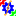icon on desktop to launch the application.

3. Pin (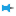) the Explorer dock on the right and the Toolbox dock on the left.

4. Click the second icon within the Explorer dock,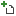to open the project. This opens the file bowser within the Explorer dock.

5. Navigate to the folder where the pre-built example project was pasted in Step 1. Double click the BuckConverter.project node within that folder to open the project.

6. Click the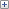icon to explore the files within the project. To display the model file, double click the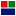Buck node in the Explorer dock.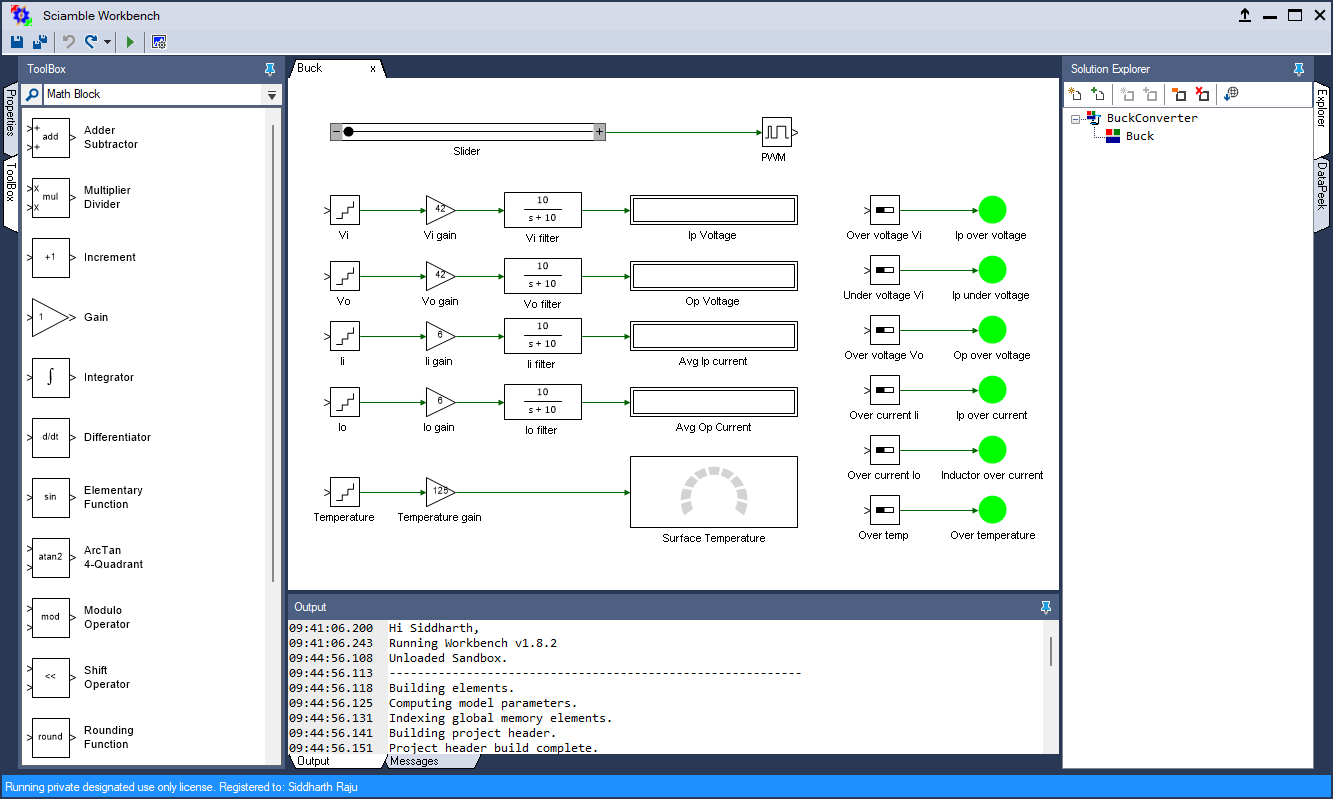7. The above model is created by dragging-and-dropping and interconnecting the tools available in the toolbox dock. The purpose of each of the tool in the model is listed in the table below:

Tool name Operation
Slider Enables varying the power-pole duty cycle from 0 to 1 in real-time.
PWM Relays the duty cycle, selected through the slider, to the PWM controller which controls the gate signal for the Si power-pole.
Voltage A Using ADC, reads the voltage across Vin+ and Vin-, and outputs the normalized value between ±1.
Voltage A gain Scales the normalized value from the ADC back to the actual value. Gain is 42 since ADC reading of 1 ≘ 42 V.
Filter Filters the Vin+ voltage measurement to remove noise. The low-pass filter bandwidth is set to 10 rad/s.
Ip Voltage Displays the filtered input voltage.
Voltage B Using ADC, reads the voltage across Vout+ and Vout-, and outputs the normalized value between ±1.
Voltage B gain Scales the normalized value from the ADC back to the actual value.
Filter1 Filters the Vout+ voltage measurement to remove noise. The low-pass filter bandwidth is set to 10 rad/s.
Op Voltage Displays the filtered output voltage.
Current A Using ADC, reads the current from the top of the power-pole into the Vin+ terminal, and outputs the normalized value between ±1.
Current A gain Scales the normalized value from the ADC back to the actual value. Gain is -6 since ADC reading of 1 ≘ 6 A, and is negative since it is preferred to have positive value for current flowing out of the power supply's positive (Vin+) terminal.
Sensor offset The Hall-effect current sensors on the lab kits develop offset during regular operation and must be compensated for during each lab for proper measurment. This tool removes the pre-existing offset in Current A sensor, if any.
Filter2 Filters the Current A measurement to remove both noise and switching ripple content. The low-pass filter bandwidth is set to 10 rad/s. High frequency components such as ripple in the current cannot be observed using the Workbench scope due to limited bandwidth of the USB connection. Hence a DSO must be used for observing switching frequency components.
Avg Ip Current Displays the filtered average input current.
Current B Using ADC, reads the current from the Vout+ terminal into the inductor, and outputs the normalized value between ±1.
Current B gain Scales the normalized value from the ADC back to the actual value.
Sensor offset1 This tool removes the pre-existing offset in Current B sensor, if any.
Filter3 Filters the Current B measurement to remove both noise and switching ripple content. The low-pass filter bandwidth is set to 10 rad/s.
Avg Op Current Displays the filtered average inductor/output current.
Temperature Using ADC, reads the temperature at the device-heatsink junction, and outputs the normalized value between ±1.
Temperatur gain Scales the normalized value from the ADC back to the actual value. Gain is 125 since ADC reading of 1 ≘ 125 °C.
Surface temperature Displays the instantaenous device-heatsink junction temperature.
Over voltage A Status flag that outputs True when the instantaenous voltage across Vin+ and Vin- exceeds 25 V.
Under voltage A Status flag that outputs True when the instantaenous voltage across Vin+ and Vin- falls below 14 V.
Over voltage B Status flag that outputs True when the instantaenous voltage across Vout+ and Vout- exceeds 25 V.
Over current A Status flag that outputs True when the instantaenous current from Vin+ terminal exceeds ±4 A.
Over current B Status flag that outputs True when the instantaenous inductor current exceeds ±4 A.
Over temp Status flag that outputs True when the instantaenous device-heatsink junction temperature exceeds 85 °C.
Input over voltage Green indicates that the input voltage is less than 25 V, i.e., the Over voltage A status flag is false. Else, will turn Red if Over voltage A status flag is true.
Input under voltage Green indicates that the input voltage is greater than 14 V, i.e., the Under voltage A status flag is false. Else, will turn Red if Under voltage A status flag is true.
Output over voltage Green indicates that the output voltage is less than 25 V, i.e., the Over voltage B status flag is false. Else, will turn Red if Over voltage B status flag is true.
Input over current Green indicates that the input current is less than ±4 A, i.e., the Over current A status flag is false. Else, will turn Red if Over current A status flag is true.
Inductor over current Green indicates that the inductor current is less than ±4 A, i.e., the Over current B
Over temperature Green indicates that the device-heatsink junction temperature is less than 85 °C, i.e., the Over temp status flag is false. Else, will turn Red if Over temp status flag is true.

8. Ensure that the switching frequency is set to 100 kHz. To do this, double click on the project node,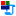BuckConverter, in the Explorer. This should bring up the project properties in the Properties dock on the left.Go to the next page by clicking the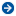in the property dock or choosing Device configuration from the drop-down menu. Check that the Frequency within PWM Configuration is set to 100000.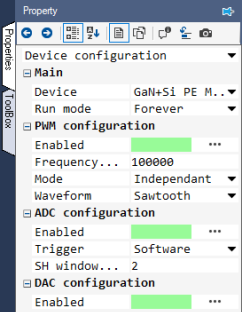##### Preparing the setup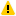Warning

Before proceeding, ensure that the isolated power supply is powered down and that the USB cable is disconnected.

1. Magnetics card connection: Unscrew any existing magnetics card and replace it with the Buck/Boost/Buck-boost magnetics card. Prior to connecting the card, ensure that the jumper on the bottom of the magnetics card is removed. Make sure that all the 6-pins of the magnetics card are making solid contact with the power-pole board.Warning

Never leave the magnetics card unscrewed. If contact is lost while the converter is running, it has the potential to cause very high voltage due to interruption of inductor current, potentially damaging the converter, or worse, could lead to a safety hazard.

2. Rheostat setting: Set the slider such that the resistance across the two closest rheostat terminals is 10 Ω.

3. Power connections:

1. Connect the Vin terminals to the isolated power supply:

1. DC +ve: Vin+ (Red)

2. Ground: Earth (Green)

3. `DC −ve: ● Vin− (Black)`

2. Connect the Vo terminals to the rheostat load:

1. Rheostat rail: Vo+ (Red)

2. `Rheostat's other terminal that is closest to the rail: ● Vo− (Black)`

4. DSO connections:

1. Connect DSO channel 1 probe to Io. Set the following options, if they are supported by the DSO:

1. Set the probe to 1x.

2. Set the measurement type as Current.

3. Set the scaling factor to 5x.

4. Set the offset at 1.5 V or 7.5 A.

5. Set termination to 1 MHz.

6. Set the channel as inverted.

2. Connect DSO channel 2 probe to SWA. Set the following options, if they are supported by the DSO:

1. Set the probe to 1x.

2. Set the measurement type as Voltage.

3. Set the scaling factor to 1x.

4. Set the offset at 0 V.

5. Set termination to 1 MHz.

6. Set the channel as default/non-inverted.

3. Connect DSO channel 3 probe to Iin. Use the same setting as that of Channel 1.

4. Connect DSO channel 4 probe to Vin. Use the same setting as that of Channel 2.

5. Jumper settings:

1. Insert the Vdc jumper, that connects the drain of the top switches to the positive DC bus, Vin+.

2. Insert the GaN/Si jumper to the Si side. This connects the buck converter inductor to the mid point of the Si power pole.

3. Insert the GaN switch's external diode jumper, labelled "Diode". Unlike the Si power MOSFET, the GaN FET does not have an intrinsic body diode. Hence a schottky diode is connected in parallel.

4. The jumper on the bottom of the magnetics card was removed in Step 1, while inserting the card.

6. Connect the USB cable to the power-pole board and the computer.

7. DC power supply settings:

1. Make sure that the DC power supply is fully turned down to 0 V prior to turning on the supply.

2. Turn on the power supply and gradually ramp up the voltage from 0 V to 15 V.

3. If the option is available, set the power supply current limit at 4.5 A.

The final wiring should look similar to this: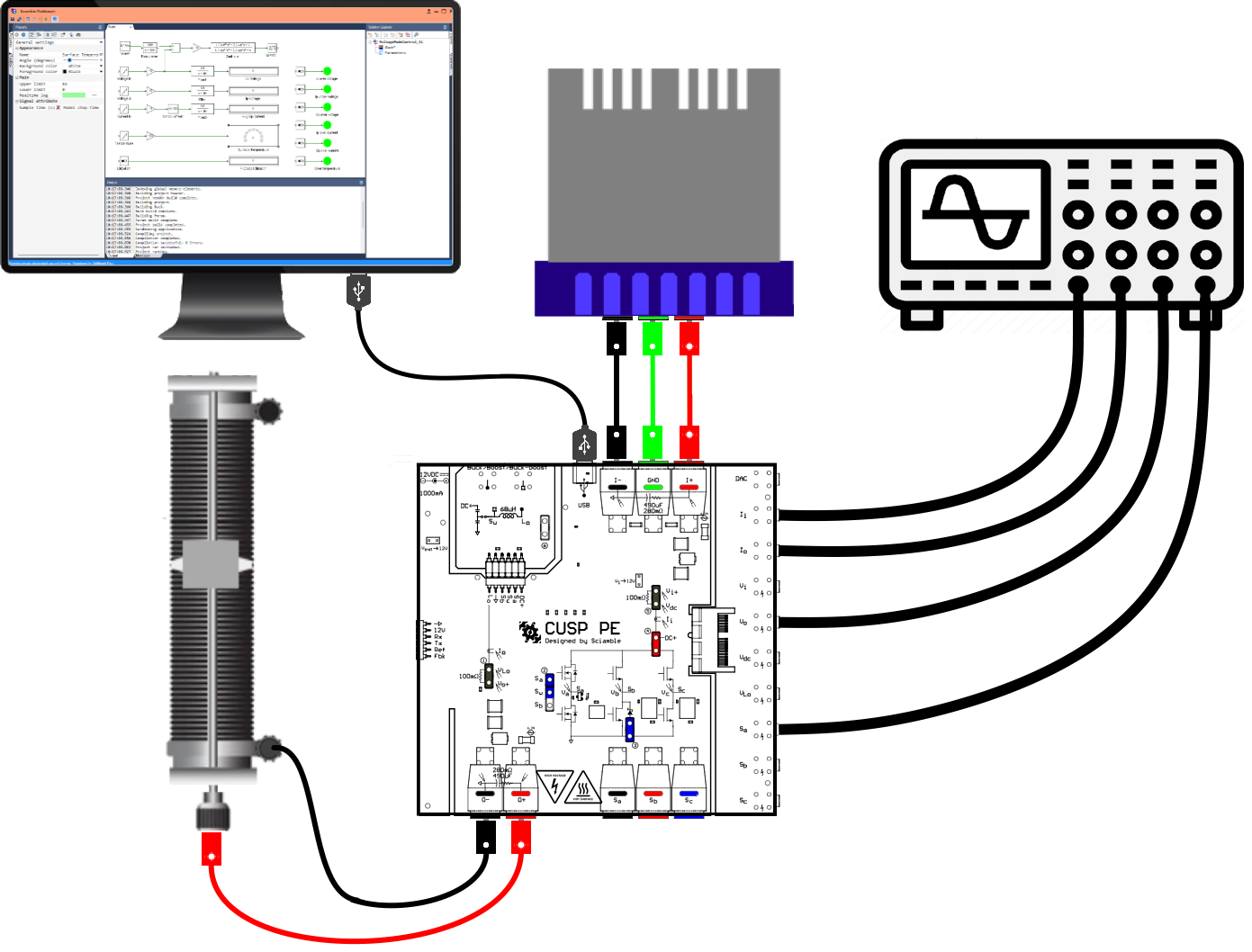##### Real-time switching characteristics measurement for Si MOSFET and diode
Running the setup:
1. Click on the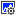icon in the top dock of Workbench to transition from the simulation mode to the real-time mode.

2. Click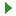to run the control algorithm in real-time.

3. If an undervoltage fault occurs, slightly increase the input voltage above 15 V but less than 16 V. Stop the model by clicking on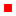and rerun it.

4. Compensate the offset in the current sensors. To do this, make note of the value, up to 3 decimal places, displayed on the Avg Ip Current and the Avg Op Current tool.

5. Click onto stop the model.

6. Open Sensor offset tool's property by double clicking on it and set the Offset value to the negative of the measured Avg Ip Current in the previous step.

Open Sensor offset1 tool's property and set the Offset value to the negative of the measured Avg Op Current in the previous step.

7. Clickto rerun model.

8. Once programmed, ensure that the Avg Ip Current and the Avg Op Current both initally display a value of 0 for up to 2 decimal places. If not, repeat the previous 3 steps.

9. Gradually increment the duty cycle from 0 to 0.5. If a fault occurs, stop the model by clicking onbutton and re-run the model.

10. Make the following measurements:.

1. Observe and make a copy of the voltage across the diode (channel 2). Adjust the time base to show the switching details during turn-ON and turn-OFF.

Measure the forward voltage drop across the diode.

2. Observe the DSO waveforms and make a copy of the voltage across the MOSFET (channel 4 - channel 2). To do this, use the DSO's math operation to get the difference between the input voltage (channel 4) and the diode voltage (channel 2).

Measure the forward voltage drop across the MOSFET during the ON period.

3. Measure the average load current Io (channel 1), and the duty cycle of operation.

##### Real-time switching characteristics measurement for GaN FET and diode
Running the setup:
1. Stop the model by clicking on.

2. Turn OFF the DC power supply and disconnect the USB cable.

3. Change the PWM channel from Si to GaN switch. To do this, open the property of the tool labelled PWM in the model by double clicking on it. Change the Channel from 1 to 3.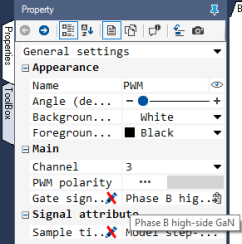4. DSO setting: Connect DSO channel 2 probe to SWB instead of SWA. This measures the voltage at the mid point of the GaN power pole with respect to Vin-.

5. Jumper setting: Change the GaN/Si jumper to the GaN side. This connects the buck converter inductor to the mid point of the GaN power pole.

6. Reconnect the USB cable.

7. Turn on the power supply and set the voltage to 15 V.

8. Clickto run the control algorithm in real-time.

9. Once programmed, gradually increment the duty cycle from 0 to 0.5.

10. Repeat the earlier measurements made for the Si power pole.

11. Click onto stop the model.

12. Turn OFF the power supply and disconnect the USB cable.

##### Lab report and reading assignment
1. Attach the DSO waveforms for turn-ON and turn-OFF of the diode showing the switching details.

2. Calculate the conduction loss of the diode using Eqn. 1.

3. Attach the DSO waveforms for Si MOSFET and the GaN FET showing the switching characteristics.

4. Estimate the Rds(ON) of the Si MOSFET and the GaN FET. Rds(ON) is determined by dividing the average voltage drop across the switch by the average inductor current, both measured during the turn-ON period.

5. Calculate the conduction loss of the Si MOSFET and GaN FET using Eqn. 3.

##### Reference
1. "Power Electronics, A First Course," Ned Mohan and Siddharth Raju, Wiley Publication.

< Introduction : previous topic